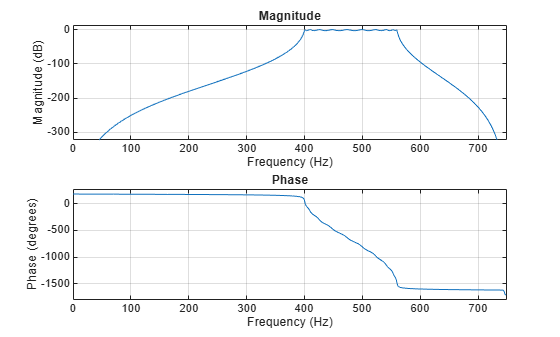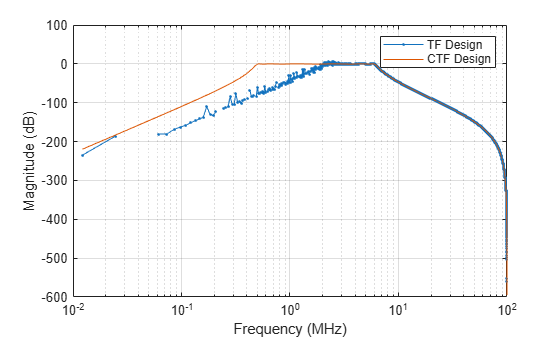# cheby1

Chebyshev Type I filter design

## Syntax

``[b,a] = cheby1(n,Rp,Wp)``
``[b,a] = cheby1(n,Rp,Wp,ftype)``
``[z,p,k] = cheby1(___)``
``[A,B,C,D] = cheby1(___)``
``[___] = cheby1(___,'s')``

## Description

example

````[b,a] = cheby1(n,Rp,Wp)` returns the transfer function coefficients of an `n`th-order lowpass digital Chebyshev Type I filter with normalized passband edge frequency `Wp` and `Rp` decibels of peak-to-peak passband ripple.```

example

````[b,a] = cheby1(n,Rp,Wp,ftype)` designs a lowpass, highpass, bandpass, or bandstop Chebyshev Type I filter, depending on the value of `ftype` and the number of elements of `Wp`. The resulting bandpass and bandstop designs are of order 2`n`.Note:   See Limitations for information about numerical issues that affect forming the transfer function.```

example

````[z,p,k] = cheby1(___)` designs a lowpass, highpass, bandpass, or bandstop digital Chebyshev Type I filter and returns its zeros, poles, and gain. This syntax can include any of the input arguments in previous syntaxes.```

example

````[A,B,C,D] = cheby1(___)` designs a lowpass, highpass, bandpass, or bandstop digital Chebyshev Type I filter and returns the matrices that specify its state-space representation.```

example

````[___] = cheby1(___,'s')` designs a lowpass, highpass, bandpass, or bandstop analog Chebyshev Type I filter with passband edge angular frequency `Wp` and `Rp` decibels of passband ripple.```

## Examples

collapse all

Design a 6th-order lowpass Chebyshev Type I filter with 10 dB of passband ripple and a passband edge frequency of 300 Hz, which, for data sampled at 1000 Hz, corresponds to $0.6\pi$ rad/sample. Plot its magnitude and phase responses. Use it to filter a 1000-sample random signal.

```[b,a] = cheby1(6,10,0.6); freqz(b,a)``````dataIn = randn(1000,1); dataOut = filter(b,a,dataIn);```

Design a 6th-order Chebyshev Type I bandstop filter with normalized edge frequencies of $0.2\pi$ and $0.6\pi$ rad/sample and 5 dB of passband ripple. Plot its magnitude and phase responses. Use it to filter random data.

```[b,a] = cheby1(3,5,[0.2 0.6],'stop'); freqz(b,a)``````dataIn = randn(1000,1); dataOut = filter(b,a,dataIn);```

Design a 9th-order highpass Chebyshev Type I filter with 0.5 dB of passband ripple and a passband edge frequency of 300 Hz, which, for data sampled at 1000 Hz, corresponds to $0.6\pi$ rad/sample. Plot the magnitude and phase responses. Convert the zeros, poles, and gain to second-order sections for use by `fvtool`.

```[z,p,k] = cheby1(9,0.5,300/500,'high'); sos = zp2sos(z,p,k); fvtool(sos,'Analysis','freq')```Design a 20th-order Chebyshev Type I bandpass filter with a lower passband frequency of 500 Hz and a higher passband frequency of 560 Hz. Specify a passband ripple of 3 dB and a sample rate of 1500 Hz. Use the state-space representation. Design an identical filter using `designfilt`.

```[A,B,C,D] = cheby1(10,3,[500 560]/750); d = designfilt('bandpassiir','FilterOrder',20, ... 'PassbandFrequency1',500,'PassbandFrequency2',560, ... 'PassbandRipple',3,'SampleRate',1500);```

Convert the state-space representation to second-order sections. Visualize the frequency responses using `fvtool`.

```sos = ss2sos(A,B,C,D); fvt = fvtool(sos,d,'Fs',1500); legend(fvt,'cheby1','designfilt')```Design a 5th-order analog Butterworth lowpass filter with a cutoff frequency of 2 GHz. Multiply by $2\pi$ to convert the frequency to radians per second. Compute the frequency response of the filter at 4096 points.

```n = 5; f = 2e9; [zb,pb,kb] = butter(n,2*pi*f,'s'); [bb,ab] = zp2tf(zb,pb,kb); [hb,wb] = freqs(bb,ab,4096);```

Design a 5th-order Chebyshev Type I filter with the same edge frequency and 3 dB of passband ripple. Compute its frequency response.

```[z1,p1,k1] = cheby1(n,3,2*pi*f,'s'); [b1,a1] = zp2tf(z1,p1,k1); [h1,w1] = freqs(b1,a1,4096);```

Design a 5th-order Chebyshev Type II filter with the same edge frequency and 30 dB of stopband attenuation. Compute its frequency response.

```[z2,p2,k2] = cheby2(n,30,2*pi*f,'s'); [b2,a2] = zp2tf(z2,p2,k2); [h2,w2] = freqs(b2,a2,4096);```

Design a 5th-order elliptic filter with the same edge frequency, 3 dB of passband ripple, and 30 dB of stopband attenuation. Compute its frequency response.

```[ze,pe,ke] = ellip(n,3,30,2*pi*f,'s'); [be,ae] = zp2tf(ze,pe,ke); [he,we] = freqs(be,ae,4096);```

Plot the attenuation in decibels. Express the frequency in gigahertz. Compare the filters.

```plot(wb/(2e9*pi),mag2db(abs(hb))) hold on plot(w1/(2e9*pi),mag2db(abs(h1))) plot(w2/(2e9*pi),mag2db(abs(h2))) plot(we/(2e9*pi),mag2db(abs(he))) axis([0 4 -40 5]) grid xlabel('Frequency (GHz)') ylabel('Attenuation (dB)') legend('butter','cheby1','cheby2','ellip')```The Butterworth and Chebyshev Type II filters have flat passbands and wide transition bands. The Chebyshev Type I and elliptic filters roll off faster but have passband ripple. The frequency input to the Chebyshev Type II design function sets the beginning of the stopband rather than the end of the passband.

## Input Arguments

collapse all

Filter order, specified as an integer scalar. For bandpass and bandstop designs, `n` represents one-half the filter order.

Data Types: `double`

Peak-to-peak passband ripple, specified as a positive scalar expressed in decibels.

If your specification, ℓ, is in linear units, you can convert it to decibels using `Rp` = 40 log10((1+ℓ)/(1–ℓ)).

Data Types: `double`

Passband edge frequency, specified as a scalar or a two-element vector. The passband edge frequency is the frequency at which the magnitude response of the filter is –`Rp` decibels. Smaller values of passband ripple, `Rp`, result in wider transition bands.

• If `Wp` is a scalar, then `cheby1` designs a lowpass or highpass filter with edge frequency `Wp`.

If `Wp` is the two-element vector `[w1 w2]`, where `w1` < `w2`, then `cheby1` designs a bandpass or bandstop filter with lower edge frequency `w1` and higher edge frequency `w2`.

• For digital filters, the passband edge frequencies must lie between 0 and 1, where 1 corresponds to the Nyquist rate—half the sample rate or π rad/sample.

For analog filters, the passband edge frequencies must be expressed in radians per second and can take on any positive value.

Data Types: `double`

Filter type, specified as one of the following:

• `'low'` specifies a lowpass filter with passband edge frequency `Wp`. `'low'` is the default for scalar `Wp`.

• `'high'` specifies a highpass filter with passband edge frequency `Wp`.

• `'bandpass'` specifies a bandpass filter of order 2`n` if `Wp` is a two-element vector. `'bandpass'` is the default when `Wp` has two elements.

• `'stop'` specifies a bandstop filter of order 2`n` if `Wp` is a two-element vector.

## Output Arguments

collapse all

Transfer function coefficients of the filter, returned as row vectors of length `n` + 1 for lowpass and highpass filters and 2`n` + 1 for bandpass and bandstop filters.

• For digital filters, the transfer function is expressed in terms of `b` and `a` as

`$H\left(z\right)=\frac{B\left(z\right)}{A\left(z\right)}=\frac{\text{b(1)}+\text{b(2)}\text{\hspace{0.17em}}{z}^{-1}+\cdots +\text{b(n+1)}\text{\hspace{0.17em}}{z}^{-n}}{\text{a(1)}+\text{a(2)}\text{\hspace{0.17em}}{z}^{-1}+\cdots +\text{a(n+1)}\text{\hspace{0.17em}}{z}^{-n}}.$`

• For analog filters, the transfer function is expressed in terms of `b` and `a` as

`$H\left(s\right)=\frac{B\left(s\right)}{A\left(s\right)}=\frac{\text{b(1)}\text{\hspace{0.17em}}{s}^{n}+\text{b(2)}\text{\hspace{0.17em}}{s}^{n-1}+\cdots +\text{b(n+1)}}{\text{a(1)}\text{\hspace{0.17em}}{s}^{n}+\text{a(2)}\text{\hspace{0.17em}}{s}^{n-1}+\cdots +\text{a(n+1)}}.$`

Data Types: `double`

Zeros, poles, and gain of the filter, returned as two column vectors of length `n` (2`n` for bandpass and bandstop designs) and a scalar.

• For digital filters, the transfer function is expressed in terms of `z`, `p`, and `k` as

`$H\left(z\right)=\text{k}\frac{\left(1-\text{z(1)}\text{\hspace{0.17em}}{z}^{-1}\right)\text{\hspace{0.17em}}\left(1-\text{z(2)}\text{\hspace{0.17em}}{z}^{-1}\right)\cdots \left(1-\text{z(n)}\text{\hspace{0.17em}}{z}^{-1}\right)}{\left(1-\text{p(1)}\text{\hspace{0.17em}}{z}^{-1}\right)\text{\hspace{0.17em}}\left(1-\text{p(2)}\text{\hspace{0.17em}}{z}^{-1}\right)\cdots \left(1-\text{p(n)}\text{\hspace{0.17em}}{z}^{-1}\right)}.$`
• For analog filters, the transfer function is expressed in terms of `z`, `p`, and `k` as

`$H\left(s\right)=\text{k}\frac{\left(s-\text{z(1)}\right)\text{\hspace{0.17em}}\left(s-\text{z(2)}\right)\cdots \left(s-\text{z(n)}\right)}{\left(s-\text{p(1)}\right)\text{\hspace{0.17em}}\left(s-\text{p(2)}\right)\cdots \left(s-\text{p(n)}\right)}.$`

Data Types: `double`

State-space representation of the filter, returned as matrices. If m = `n` for lowpass and highpass designs and m = 2`n` for bandpass and bandstop filters, then `A` is m × m, `B` is m × 1, `C` is 1 × m, and `D` is 1 × 1.

• For digital filters, the state-space matrices relate the state vector x, the input u, and the output y through

`$\begin{array}{c}x\left(k+1\right)=\text{A}\text{\hspace{0.17em}}x\left(k\right)+\text{B}\text{\hspace{0.17em}}u\left(k\right)\\ y\left(k\right)=\text{\hspace{0.17em}}\text{\hspace{0.17em}}\text{C}\text{\hspace{0.17em}}x\left(k\right)+\text{D}\text{\hspace{0.17em}}u\left(k\right).\end{array}$`

• For analog filters, the state-space matrices relate the state vector x, the input u, and the output y through

`$\begin{array}{l}\stackrel{˙}{x}=\text{A}\text{\hspace{0.17em}}x+\text{B}\text{\hspace{0.17em}}u\\ y=\text{C}\text{\hspace{0.17em}}x+\text{D}\text{\hspace{0.17em}}u.\end{array}$`

Data Types: `double`

collapse all

### Limitations

Numerical Instability of Transfer Function Syntax

In general, use the `[z,p,k]` syntax to design IIR filters. To analyze or implement your filter, you can then use the `[z,p,k]` output with `zp2sos`. If you design the filter using the `[b,a]` syntax, you might encounter numerical problems. These problems are due to round-off errors and can occur for `n` as low as 4. The following example illustrates this limitation.

```n = 6; Rp = 0.1; Wn = [2.5e6 29e6]/500e6; ftype = 'bandpass'; % Transfer function design [b,a] = cheby1(n,Rp,Wn,ftype); % This filter is unstable % Zero-pole-gain design [z,p,k] = cheby1(n,Rp,Wn,ftype); sos = zp2sos(z,p,k); % Plot and compare the results hfvt = fvtool(b,a,sos,'FrequencyScale','log'); legend(hfvt,'TF Design','ZPK Design')```## Algorithms

Chebyshev Type I filters are equiripple in the passband and monotonic in the stopband. Type I filters roll off faster than Type II filters, but at the expense of greater deviation from unity in the passband.

`cheby1` uses a five-step algorithm:

1. It finds the lowpass analog prototype poles, zeros, and gain using the function `cheb1ap`.

2. It converts the poles, zeros, and gain into state-space form.

3. If required, it uses a state-space transformation to convert the lowpass filter to a highpass, bandpass, or bandstop filter with the desired frequency constraints.

4. For digital filter design, it uses `bilinear` to convert the analog filter into a digital filter through a bilinear transformation with frequency prewarping. Careful frequency adjustment enables the analog filters and the digital filters to have the same frequency response magnitude at `Wp` or `w1` and `w2`.

5. It converts the state-space filter back to transfer function or zero-pole-gain form, as required.